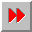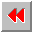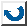EMA-XPS Online

EQUAL

```EQUAL
=====

syntax: (equal <object1> <object2>)   ; Common Lisp
(is-same <object1> <object2>) ; Babylon Lisp

This function compares both objects. It returns true,
when the objects are structurally similar. Numbers
(equal works like '=') and characters will be compared
like eql would do. Symbols will be compared like eq
would do.

example:       >(equal 1 1.0)
NIL
>(equal #\a #\a)
T
>(equal #\a #\A)
NIL

```EMA-XPS Online